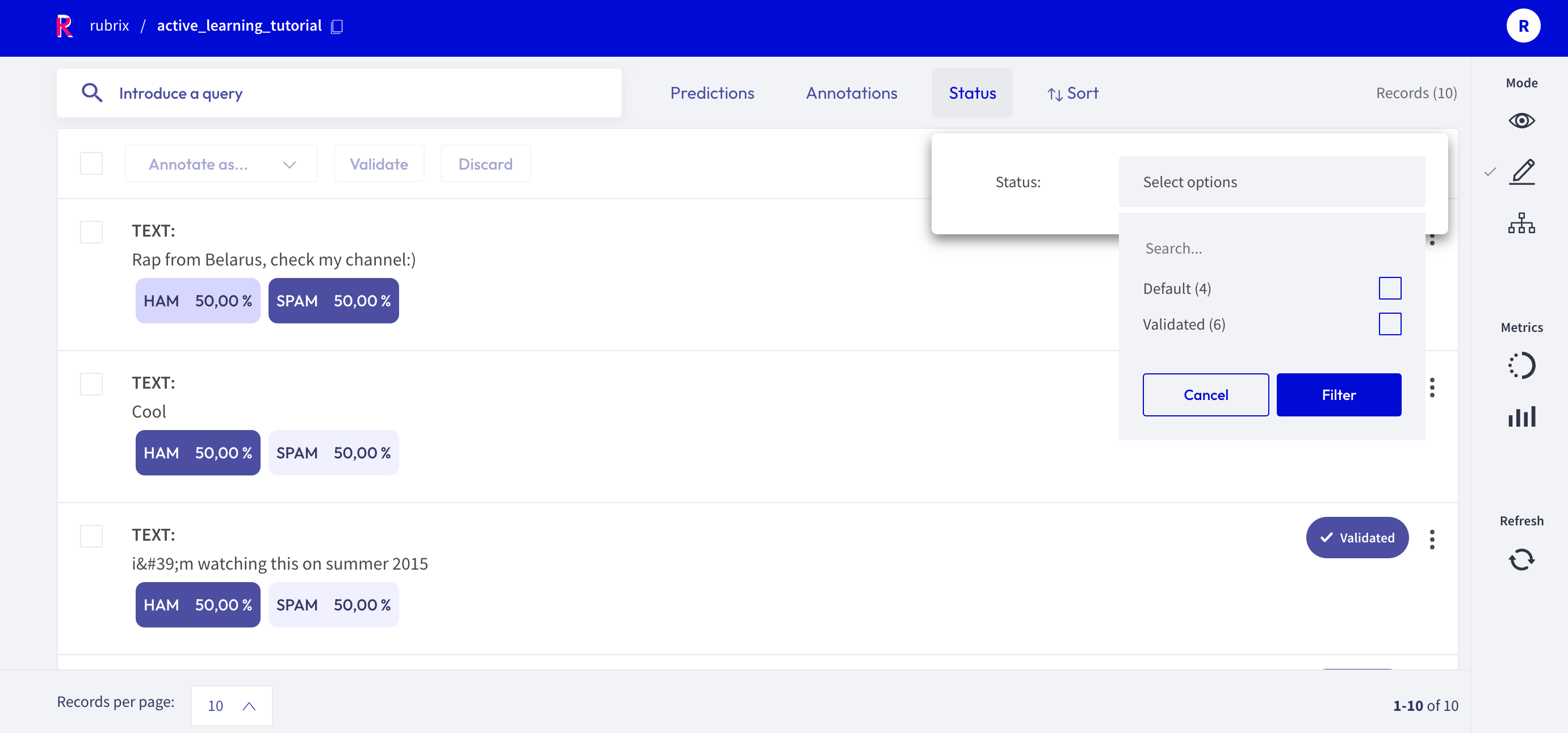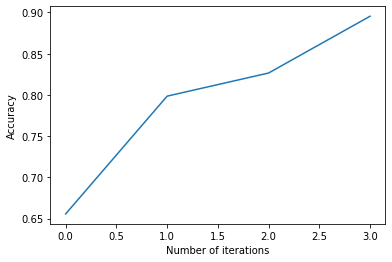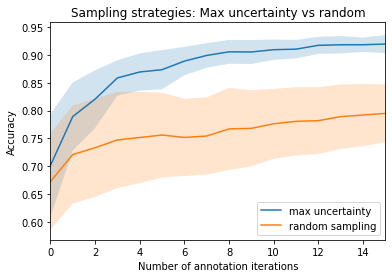# 🤔 Active learning with ModAL and scikit-learn#

In this tutorial, we will walk through the process of building an active learning prototype with Rubrix, ModAL and scikit-learn.

• 💻 We train a spam filter using the YouTube Spam Collection data set.

• 🎩 For this we embed a lightweight scikit-learn classifier in an active learner via ModAL.

• 🏆 We design an active learning loop around Rubrix, to quickly build up a training data set from scratch.

## Introduction#

Active learning is a special case of machine learning in which a learning algorithm can interactively query a user (or some other information source) to label new data points with the desired outputs. In statistics literature, it is sometimes also called optimal experimental design. The information source is also called teacher or oracle. [Wikipedia]

In this tutorial our goal is to show you how to incorporate Rubrix into an active learning workflow involving a human in the loop. We will build a simple text classifier by combining scikit-learn, the active learning framework ModAL and Rubrix. Scikit-learn will provide the model that we will embed in an active learner from ModAL, and you and Rubrix will serve as the information source that teach the model to become a sample efficient classifier.

This tutorial is only a proof of concept for educational purposes and to inspire you with some ideas involving interactive learning processes, and how they can help to quickly build a training data set from scratch.

## Setup#

Rubrix, is a free and open-source tool to explore, annotate, and monitor data for NLP projects.

If you are new to Rubrix, check out the ⭐ Github repository.

If you have not installed and launched Rubrix yet, check the Setup and Installation guide.

For this tutorial we also need the third party libraries modAl, scikit-learn and matplotlib (optional), which can be installed via pip:

[ ]:

%pip install modAL scikit-learn matplotlib -qqq  # matplotlib is optional


Rubrix allows you to log and track data for different NLP tasks, such as Token Classification or Text Classification.

In this tutorial, we will use the YouTube Spam Collection dataset, which is a binary classification task for detecting spam comments in YouTube videos.

Let’s load the data and have a look at it:

:

import pandas as pd


:

test_df

:

COMMENT_ID AUTHOR DATE CONTENT CLASS VIDEO
0 z120djlhizeksdulo23mj5z52vjmxlhrk04 Murlock Nightcrawler 2015-05-24T07:04:29.844000 Charlie from LOST?﻿ 0 3
1 z133ibkihkmaj3bfq22rilaxmp2yt54nb Debora Favacho (Debora Sparkle) 2015-05-21T14:08:41.338000 BEST SONG EVER X3333333333﻿ 0 4
2 z12gxdortqzwhhqas04cfjrwituzghb5tvk0k Muhammad Asim Mansha NaN Aslamu Lykum... From Pakistan﻿ 1 3
3 _2viQ_Qnc6_ZYkMn1fS805Z6oy8ImeO6pSjMLAlwYfM mile panika 2013-11-03T14:39:42.248000 I absolutely adore watching football plus I’ve... 1 4
4 z120s1agtmmetler404cifqbxzvdx15idtw0k Sheila Cenabre 2014-08-19T12:33:11 I really love this video.. http://www.bubblews... 1 1
... ... ... ... ... ... ...
387 z13pup2w2k3rz1lxl04cf1a5qzavgvv51vg0k geraldine lopez 2015-05-20T23:44:25.920000 love the you lie the good﻿ 0 3
388 z13psdarpuzbjp1hh04cjfwgzonextlhf1w bilal bilo 2015-05-22T20:36:36.926000 I liked<br />﻿ 0 4
389 z131xnwierifxxkj204cgvjxyo3oydb42r40k YULIOR ZAMORA 2014-09-10T01:35:54 I loved it so much ... 0 1
390 z12pwrxj0kfrwnxye04cjxtqntycd1yia44 ‫ארז אריה‬‎ 2015-05-15T19:46:53.719000 good party﻿ 0 2
391 z13oxvzqrzvyit00322jwtjo2tzqylhof04 Octavia W 2015-05-22T02:33:26.041000 Waka waka ﻿ 0 4

392 rows × 6 columns

As we can see, the data contains the comment id, the author of the comment, the date, the content (the comment itself) and a class column that indicates if a comment is spam or ham. We will use the class column only in the test dataset to illustrate the effectiveness of the active learning approach with Rubrix. For the training dataset, we will simply ignore the column and assume that we are gathering training data from scratch.

## 2. Defining our classifier and Active Learner#

In this tutorial, we will use a multinomial Naive Bayes classifier that is suitable for classification with discrete features (e.g., word counts for text classification):

:

from sklearn.naive_bayes import MultinomialNB

# Define our classification model
classifier = MultinomialNB()


Then, we will define our active learner, which uses the classifier as an estimator of the most uncertain predictions:

:

from modAL.models import ActiveLearner

# Define active learner
learner = ActiveLearner(
estimator=classifier,
)


The features for our classifier will be the counts of different word n-grams: that is, for each example we count the number of contiguous sequences of n words, where n goes from 1 to 5.

The output of this operation will be matrices of n-gram counts for our train and test data set, where each element in a row equals the counts of a specific word n-gram found in the example:

:

from sklearn.feature_extraction.text import CountVectorizer

# The resulting matrices will have the shape of (nr of examples, nr of word n-grams)
vectorizer = CountVectorizer(ngram_range=(1, 5))

X_train = vectorizer.fit_transform(train_df.CONTENT)
X_test = vectorizer.transform(test_df.CONTENT)


## 3. Active Learning loop#

Now we can start our active learning loop that consists of iterating over following steps:

1. Annotate samples

2. Teach the active learner

3. Plot the improvement (optional)

Before starting the learning loop, let us define two variables:

• the number of instances we want to annotate per iteration,

• a variable to keep track of our improvements by recording the achieved accuracy after each iteration.

:

# Number of instances we want to annotate per iteration
n_instances = 10

# Accuracies after each iteration to keep track of our improvement
accuracies = []


### Step 1: Annotate samples#

The first step of the training loop is about annotating n examples having the most uncertain prediction. In the first iteration, these will be just random examples, since the classifier is still not trained and we do not have predictions yet.

:

from sklearn.exceptions import NotFittedError

# query examples from our training pool with the most uncertain prediction
query_idx, query_inst = learner.query(X_train, n_instances=n_instances)

# get predictions for the queried examples
try:
probabilities = learner.predict_proba(X_train[query_idx])
# For the very first query we do not have any predictions
except NotFittedError:
probabilities = [[0.5, 0.5]]*n_instances

[ ]:

import rubrix as rb

# Build the Rubrix records
records = [
rb.TextClassificationRecord(
id=idx,
text=train_df.CONTENT.iloc[idx],
prediction=list(zip(["HAM", "SPAM"], probs)),
prediction_agent="MultinomialNB",
)
for idx, probs in zip(query_idx, probabilities)
]

# Log the records
rb.log(records, name="active_learning_tutorial")


After logging the records to Rubrix, we switch over to the UI, where we can find the newly logged examples in the active_learning_tutorial dataset.

To only show the examples that are still missing an annotation, you can select “Default” in the Status filter as shown in the screenshot below. After annotating a few examples you can press the Refresh button in the left side bar, to update the view with respect to the filters.Once you are done annotating the examples, you can continue with the active learning loop. If your annotations only contained one class, consider increasing the n_instances parameter.

### Step 2: Teach the learner#

The second step in the loop is to teach the learner. Once we have trained our classifier with the newly annotated examples, we can apply the classifier to the test data and record the accuracy to keep track of our improvement.

[ ]:

# Load the annotated records into a pandas DataFrame

# check if all examples were annotated
if any(records_df.annotation.isna()):

# train the classifier with the newly annotated examples
y_train = records_df.annotation.map(lambda x: int(x == "SPAM"))
learner.teach(X=X_train[query_idx], y=y_train.to_list())

# Keep track of our improvement
accuracies.append(learner.score(X=X_test, y=test_df.CLASS))


Now go back to step 1 and repeat both steps a couple of times.

### Step 3. Plot the improvement (optional)#

After a few iterations, we can check the current performance of our classifier by plotting the accuracies. If you think the performance can still be improved, you can repeat step 1 and 2 and check the accuracy again.

:

import matplotlib.pyplot as plt

# Plot the accuracy versus the iteration number
plt.plot(accuracies)
plt.xlabel("Number of iterations")
plt.ylabel("Accuracy");## Summary#

In this tutorial we saw how to embed Rubrix in an active learning loop, and also how it can help you to gather a sample efficient data set by annotating only the most decisive examples. We created a rather minimalist active learning loop, but Rubrix does not really care about the complexity of the loop. It will always help you to record and annotate data examples with their model predictions, allowing you to quickly build up a data set from scratch.

## Next steps#

📚 Rubrix documentation for more guides and tutorials.

🙋‍♀️ Join the Rubrix community! A good place to start is the discussion forum.

## Bonus: Compare query strategies, random vs max uncertainty#

In this bonus, we quickly demonstrate the effectiveness of annotating only the most uncertain predictions compared to random annotations. So the next time you want to build a data set from scratch, keep this strategy in mind and maybe use Rubrix for the annotation process! 😀

[ ]:

import numpy as np

n_iterations = 150
n_instances = 10
random_samples = 50

# max uncertainty strategy
accuracies_max = []
for i in range(random_samples):
train_rnd_df = train_df#.sample(frac=1)
test_rnd_df = test_df#.sample(frac=1)
X_rnd_train = vectorizer.transform(train_rnd_df.CONTENT)
X_rnd_test = vectorizer.transform(test_rnd_df.CONTENT)

accuracies, learner = [], ActiveLearner(estimator=MultinomialNB())

for i in range(n_iterations):
query_idx, _ = learner.query(X_rnd_train, n_instances=n_instances)
learner.teach(X=X_rnd_train[query_idx], y=train_rnd_df.CLASS.iloc[query_idx].to_list())
accuracies.append(learner.score(X=X_rnd_test, y=test_rnd_df.CLASS))
accuracies_max.append(accuracies)

# random strategy
accuracies_rnd = []
for i in range(random_samples):
accuracies, learner = [], ActiveLearner(estimator=MultinomialNB())

for random_idx in np.random.choice(X_train.shape, size=(n_iterations, n_instances), replace=False):
learner.teach(X=X_train[random_idx], y=train_df.CLASS.iloc[random_idx].to_list())
accuracies.append(learner.score(X=X_test, y=test_df.CLASS))
accuracies_rnd.append(accuracies)

arr_max, arr_rnd = np.array(accuracies_max), np.array(accuracies_rnd)

[ ]:

plt.plot(range(n_iterations), arr_max.mean(0))
plt.fill_between(range(n_iterations), arr_max.mean(0)-arr_max.std(0), arr_max.mean(0)+arr_max.std(0), alpha=0.2)
plt.plot(range(n_iterations), arr_rnd.mean(0))
plt.fill_between(range(n_iterations), arr_rnd.mean(0)-arr_rnd.std(0), arr_rnd.mean(0)+arr_rnd.std(0), alpha=0.2)

plt.xlim(0,15)
plt.title("Sampling strategies: Max uncertainty vs random")
plt.xlabel("Number of annotation iterations")
plt.ylabel("Accuracy")
plt.legend(["max uncertainty", "random sampling"], loc=4)

<matplotlib.legend.Legend at 0x7fa38aaaab20>## Appendix: How did we obtain the train/test data?#

[ ]:

import pandas as pd
from urllib import request
from sklearn.model_selection import train_test_split
from pathlib import Path
from tempfile import TemporaryDirectory

"""
and returns the data as a tuple with a train and test DataFrame.
"""
], None

with TemporaryDirectory() as tmpdirname:
dfs = []
file = Path(tmpdirname) / f"{i}.csv"